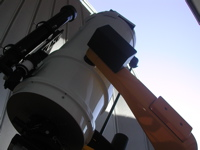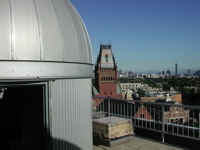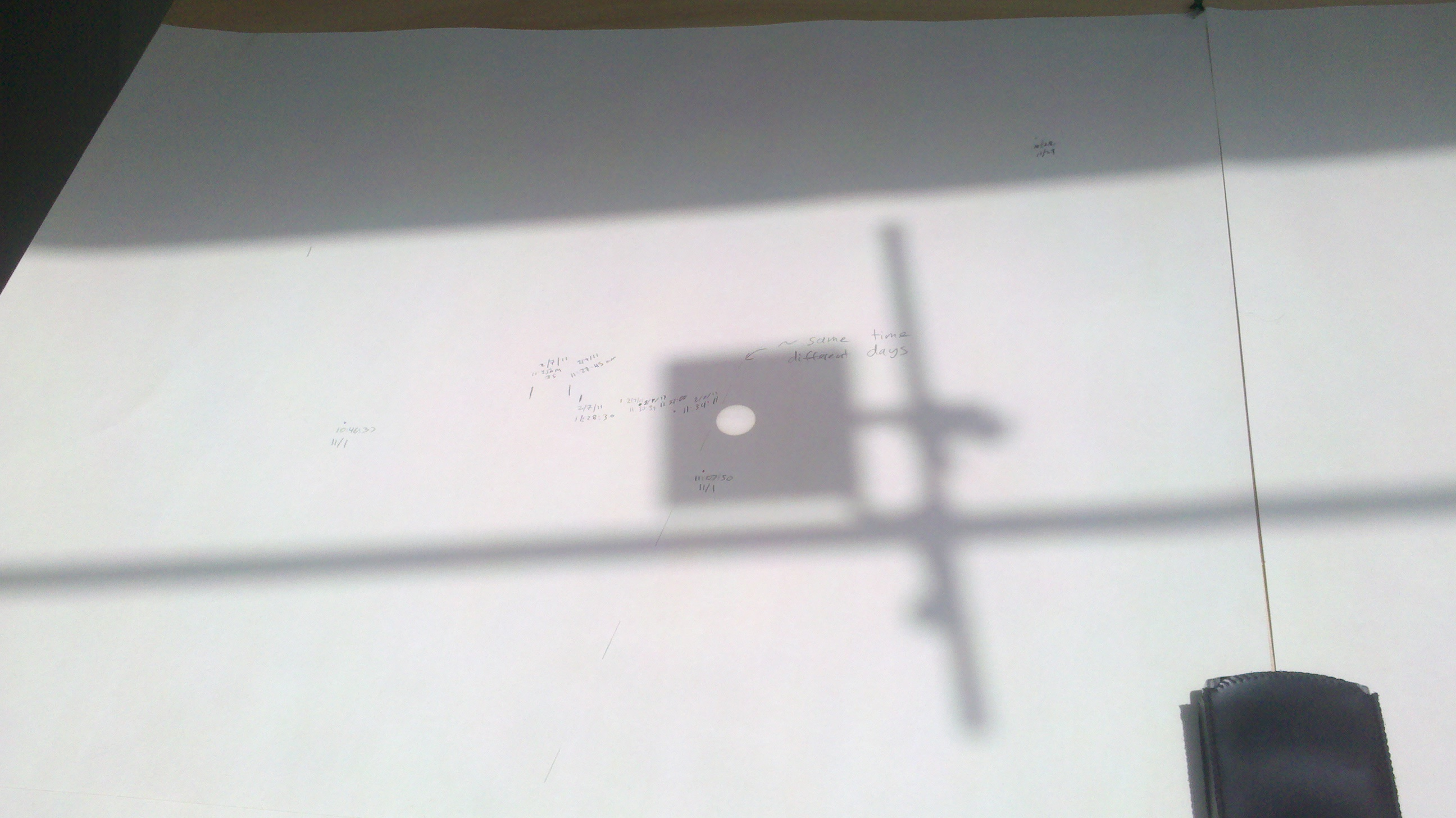# Astronomy Lab and Clay TelescopeThe Changing Declination of the Sun in the Sky

DL-Obs2

SPU-21 Fall 2015

printer version

This lab will measure the daily change in elevation above the horizon if it were measured at exactly noon. Combined with the latitude of Cambridge, this give us the solar Declination, or celestial latitude, which changes with the season with a max/min of ~ +/- 23.5 degrees on the Solstices. If we measure the rate of change (degrees per day) we can attempt to predict the solar Declination on the Winter (or Summer) solstice, which is also the same number as the tilt of the Earth's axis of rotation with respect to the plane of the Earth's orbit around the sun. This angle is the Obliquity of the Ecliptic.

Procedure:• The sunlight shines through the iris (which is adjustable) and creates a small round spot of light on the paper. Mark the center of the projection of the Sun on the easel. Be sure to mark the exact time and date on the easel.
• Repeat this a few times each lab session and at the end of each session draw a straight line through all the points (the Sun's daily motion) to create a Sun track.
• Repeat this over the course of a few weeks.

Analysis:

• You should now have multiple Sun tracks on the easel, which will span at least 3-4 weeks. We will use these to determine the daily rate at which the Sun changes in declination.
• A good way to convert the distance you measure (in cm) into the change in declination (measured in degrees) is to use the measurement of the angular diameter of the Sun as a scale factor.
• We know the Sun's angular diameter is ~0.5 degrees.
• Measure the projected image of the Sun on the easel (in cm).
• We can now derive the scale factor 1cm = XX degrees.
• Make multiple measurements, at various times, of the difference in horizontal Sun track lines (measure this difference VERTICALLY on the easel) and the uncertainty in your measurement.
• Enter them in the table (pdf or docx).
• Convert the measurements to degrees and divide by the time difference (between the two lines you measured) in days to get a more precise estimate of the daily motion in declination.
• The plot in Figure 1 relates the change of Solar declination per day vs. the angle of the Earth's rotation axis to the ecliptic plane (or Obliquity). Use your above measurement of the difference in horizontal track lines (in degrees) and Figure 1 to determine the Obliquity of the Earth (in degrees) and its uncertainty
• How does the change in declination affect the seasons?